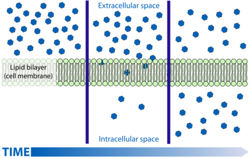# Diffusion - Physiology

## Introduction

Gases or liquids can be unevenly distributed between two areas. If one area has a higher concentration than the other then the differance between these two areas is termed the concentration gradient. The equality is then corrected by the movement of the molecules down this so called gradient from the region of high concentration to that of low. This process is passive as the molecules do not have to be forced to do this and it is reffered to as diffusion.

This works by the random thermal movement of molecules. If there is a gas present in an air tight room and then a door is opened into the next room where a lower concentration of the same gas is present the laws of probability state that more of the randomly moving molecules will escape through the door from the area of high concentration than will escape back through the door from the area of low and that eventually the concentrations in both rooms with be approximately the same. The net movement therefore will be from the room with a high concentration to that of a low concentration.

## Ficks LawA Simple Schematic Diagram of Diffusion Across a Cell Membrane(Courtesy of Mariana Ruiz Villarreal)

Q = DA((C1 - C2) ÷ L)

Q = the transport rate of a substance by diffusion

D = The diffusion coefficient

A = The cross sectional area which the substance is to diffuse across

C1 C2 = The concentrations in the two areas

L = distance separating the two areas

Ficks law demonstrates that the rate of diffusion can be maximised by increasing the area over which diffusion can occur, minimising the distance it has to occur over and increasing the gradient it occurs along. In the body it tends to be the former two which are taken into account when adapting surfaces for diffusion to occur over.

## Diffusion as a Transport Mechanism

Diffusion is very efficient over short distances and does not require energy. As such it is used in a variety of body systems where gradients and membranes suited to it are found. However over large distances diffusion is very slow.

## Diffusion Through the Lipid Bilayer

• Lipid soluble substances are able to diffuse freely across the lipid bilayer of cells.
• This occurs passively down the concentration gradient.

 Tweet WikiVet® Introduction - Help WikiVet - Report a Problem